#8种常被忽视的SQL错误用法

0
0
01. 云栖社区>
2. Java架构沉思录>
3. 博客>
4. 正文

# sql语句的执行顺序:

1. FROM <left_table>

2. ON <join_condition>

3. <join_type> JOIN <right_table>

4. WHERE <where_condition>

5. GROUP BY <group_by_list>

6. HAVING <having_condition>

7. SELECT

8. DISTINCT <select_list>

9. ORDER BY <order_by_condition>

10. LIMIT <limit_number>

# 1. LIMIT 语句

``````
``````
`SELECT * FROM operation WHERE type = 'SQLStats' `
``` ```
`AND name = 'SlowLog' ORDER BY create_time LIMIT 1000, 10;`
``` ```

``````
``````
`SELECT * FROM operation WHERE type = 'SQLStats' `
``` ```
`AND name = 'SlowLog' AND create_time > '2017-03-16 14:00:00' `
``` ```
`ORDER BY create_time limit 10;`
``` ```

# 2. 隐式转换

SQL语句中查询变量和字段定义类型不匹配是另一个常见的错误。比如下面的语句：

``````
``````
`mysql> explain extended SELECT * FROM my_balance b `
``` ```
`> WHERE b.bpn = 14000000123 `
``` ```
`> AND b.isverified IS NULL ;`
``` ```
`mysql> show warnings;`
``` ```

``` ```
`| Warning | 1739 | Cannot use ref access on index 'bpn' due to type or collation conversion on field 'bpn'`
``` ```

# 3. 关联更新、删除

``````
``````
`UPDATE operation o SET status = 'applying' WHERE o.id `
``` ```
`IN (SELECT id FROM (SELECT o.id,o.status FROM operation o `
``` ```
`WHERE o.group = 123 AND o.status NOT IN ( 'done' ) `
``` ```
`ORDER BY o.parent, o.id LIMIT 1) t);`
``` ```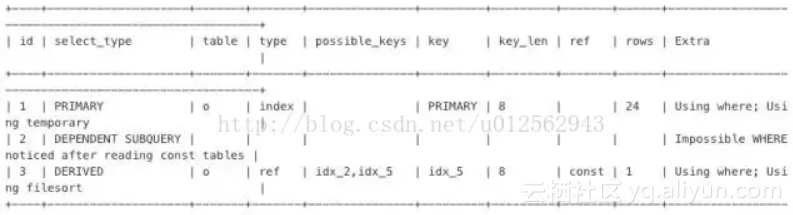``````
``````
`UPDATE operation o JOIN (SELECT o.id, o.status FROM operation o WHERE o.group = 123 `
``` ```
`AND o.status NOT IN ( 'done' ) ORDER BY o.parent,o.id LIMIT 1) t`
``` ```
`ON o.id = t.id SET status = 'applying'`
``` ```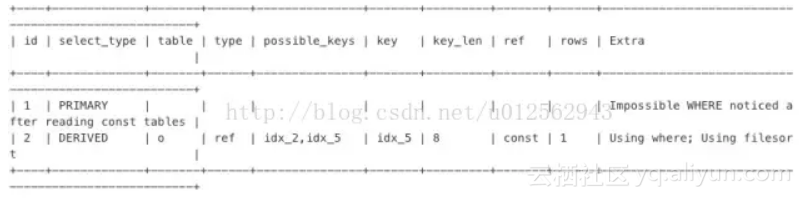# 4. 混合排序

MySQL 不能利用索引进行混合排序。但在某些场景，还是有机会使用特殊方法提升性能的。

``````
``````
`SELECT * FROM my_order o INNER JOIN my_appraise a ON a.orderid = o.id `
``` ```
`ORDER BY a.is_reply ASC, a.appraise_time DESC LIMIT 0, 20`
``` ```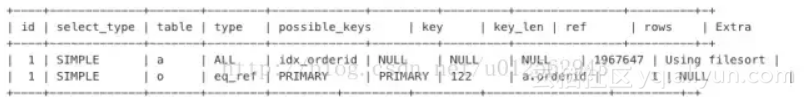``````
``````
`SELECT * FROM (`
``` ```
`(SELECT * FROM my_order o INNER JOIN my_appraise a ON a.orderid = o.id`
``` ```
`AND is_reply = 0 ORDER BY appraise_time DESC LIMIT 0, 20) `
``` ```

``` ```
`UNION ALL `
``` ```
`(SELECT * FROM my_order o INNER JOIN my_appraise a ON a.orderid = o.id`
``` ```
`AND is_reply = 1 ORDER BY appraise_time DESC LIMIT 0, 20)) t `
``` ```
`ORDER BY is_reply ASC, appraisetime DESC LIMIT 20;`
``` ```

# 5. EXISTS语句

MySQL 对待 EXISTS 子句时，仍然采用嵌套子查询的执行方式。如下面的 SQL 语句：

``````
``````
`SELECT *`
``` ```
`FROM my_neighbor n `
``` ```
`LEFT JOIN my_neighbor_apply sra `
``` ```
`ON n.id = sra.neighbor_id `
``` ```
`AND sra.user_id = 'xxx' `
``` ```
`WHERE n.topic_status < 4 `
``` ```
`AND EXISTS(SELECT 1 `
``` ```
`FROM message_info m `
``` ```
`WHERE n.id = m.neighbor_id `
``` ```
`AND m.inuser = 'xxx') `
``` ```
`AND n.topic_type <> 5`
``` ```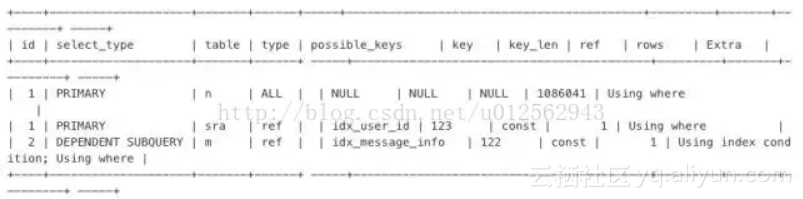``````
``````
`SELECT *`
``` ```
`FROM my_neighbor n `
``` ```
`INNER JOIN message_info m `
``` ```
`ON n.id = m.neighbor_id `
``` ```
`AND m.inuser = 'xxx' `
``` ```
`LEFT JOIN my_neighbor_apply sra `
``` ```
`ON n.id = sra.neighbor_id `
``` ```
`AND sra.user_id = 'xxx' `
``` ```
`WHERE n.topic_status < 4 `
``` ```
`AND n.topic_type <> 5`
``` ```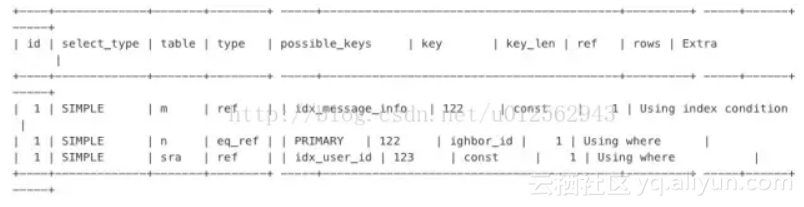6. 条件下推

1、聚合子查询；

2、含有 LIMIT 的子查询；

3、UNION 或 UNION ALL 子查询；

4、输出字段中的子查询；

``````
``````
`SELECT * `
``` ```
`FROM (SELECT target, `
``` ```
`Count(*) `
``` ```
`FROM operation `
``` ```
`GROUP BY target) t `
``` ```
`WHERE target = 'rm-xxxx'`
``` ```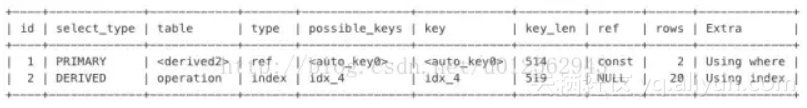``````
``````
`SELECT target, `
``` ```
`Count(*) `
``` ```
`FROM operation `
``` ```
`WHERE target = 'rm-xxxx' `
``` ```
`GROUP BY target`
``` ```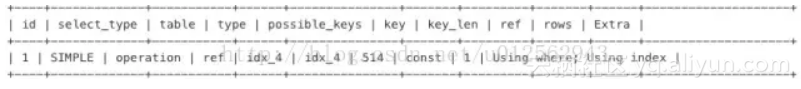# 7. 提前缩小范围

``````
``````
`SELECT * `
``` ```
`FROM my_order o `
``` ```
`LEFT JOIN my_userinfo u `
``` ```
`ON o.uid = u.uid`
``` ```
`LEFT JOIN my_productinfo p `
``` ```
`ON o.pid = p.pid `
``` ```
`WHERE ( o.display = 0 ) `
``` ```
`AND ( o.ostaus = 1 ) `
``` ```
`ORDER BY o.selltime DESC `
``` ```
`LIMIT 0, 15`
``` ```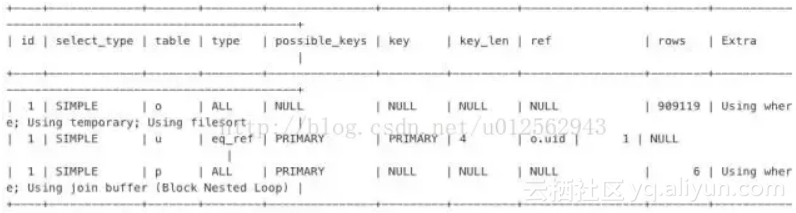``````
``````
`SELECT * `
``` ```
`FROM (`
``` ```
`SELECT * `
``` ```
`FROM my_order o `
``` ```
`WHERE ( o.display = 0 ) `
``` ```
`AND ( o.ostaus = 1 ) `
``` ```
`ORDER BY o.selltime DESC `
``` ```
`LIMIT 0, 15`
``` ```
`) o `
``` ```
`LEFT JOIN my_userinfo u `
``` ```
`ON o.uid = u.uid `
``` ```
`LEFT JOIN my_productinfo p `
``` ```
`ON o.pid = p.pid `
``` ```
`ORDER BY o.selltime DESC`
``` ```
`limit 0, 15`
``` ```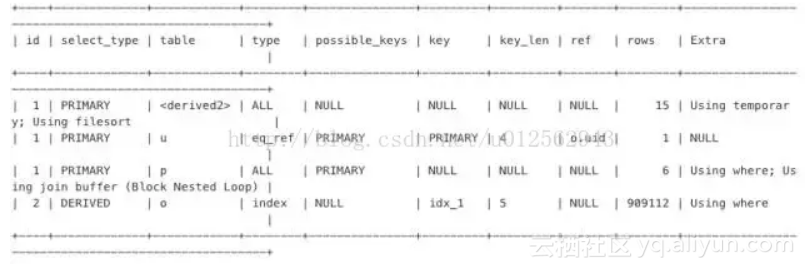8. 中间结果集下推

``````
``````
`SELECT a.*, `
``` ```
`c.allocated `
``` ```
`FROM ( `
``` ```
`SELECT resourceid `
``` ```
`FROM my_distribute d `
``` ```
`WHERE isdelete = 0 `
``` ```
`AND cusmanagercode = '1234567' `
``` ```
`ORDER BY salecode limit 20) a `
``` ```
`LEFT JOIN `
``` ```
`( `
``` ```
`SELECT resourcesid， sum(ifnull(allocation, 0) * 12345) allocated `
``` ```
`FROM my_resources `
``` ```
`GROUP BY resourcesid) c `
``` ```
`ON a.resourceid = c.resourcesid`
``` ```

``````
``````
`SELECT a.*, `
``` ```
`c.allocated `
``` ```
`FROM ( `
``` ```
`SELECT resourceid `
``` ```
`FROM my_distribute d `
``` ```
`WHERE isdelete = 0 `
``` ```
`AND cusmanagercode = '1234567' `
``` ```
`ORDER BY salecode limit 20) a `
``` ```
`LEFT JOIN `
``` ```
`( `
``` ```
`SELECT resourcesid， sum(ifnull(allocation, 0) * 12345) allocated `
``` ```
`FROM my_resources r, `
``` ```
`( `
``` ```
`SELECT resourceid `
``` ```
`FROM my_distribute d `
``` ```
`WHERE isdelete = 0 `
``` ```
`AND cusmanagercode = '1234567' `
``` ```
`ORDER BY salecode limit 20) a `
``` ```
`WHERE r.resourcesid = a.resourcesid `
``` ```
`GROUP BY resourcesid) c `
``` ```
`ON a.resourceid = c.resourcesid`
``` ```

``````
``````
`WITH a AS `
``` ```
`( `
``` ```
`SELECT resourceid `
``` ```
`FROM my_distribute d `
``` ```
`WHERE isdelete = 0 `
``` ```
`AND cusmanagercode = '1234567' `
``` ```
`ORDER BY salecode limit 20)`
``` ```
`SELECT a.*, `
``` ```
`c.allocated `
``` ```
`FROM a `
``` ```
`LEFT JOIN `
``` ```
`( `
``` ```
`SELECT resourcesid， sum(ifnull(allocation, 0) * 12345) allocated `
``` ```
`FROM my_resources r, `
``` ```
`a `
``` ```
`WHERE r.resourcesid = a.resourcesid `
``` ```
`GROUP BY resourcesid) c `
``` ```
`ON a.resourceid = c.resourcesid`
``` ```

+ 关注